Balbharati Maharashtra State Board 11th Commerce Maths Solution Book Pdf Chapter 8 Linear Inequations Ex 8.1 Questions and Answers.

## Maharashtra State Board 11th Commerce Maths Solutions Chapter 8 Linear Inequations Ex 8.1

Question 1.
Write the inequations that represent the interval and state whether the interval is bounded or unbounded:
(i) [-4, $$\frac{7}{3}$$]
Solution:
[-4, $$\frac{7}{3}$$]
Here, x takes values between -4 and $$\frac{7}{3}$$ including -4 and $$\frac{7}{3}$$
∴ the required inequation is -4 ≤ x ≤ $$\frac{7}{3}$$
∴ it is a bounded (closed) interval.

(ii) (0, 0.9]
Solution:
(0, 0.9]
Here, x takes values between 0 and 0.9, including 0.9 and excluding 0.
∴ the required inequation is 0 < x ≤ 0.9
∴ it is a bounded (semi-right closed) interval.

(iii) (-∞, ∞)
Solution:
(-∞, ∞)
Here, x takes values between -∞ and ∞
∴ the required inequation is -∞ < x < ∞
∴ it is an unbounded (open) interval.

(iv) [5, ∞)
Solution:
[5, ∞)
Here, x takes values between 5 and ∞ including 5.
∴ the required inequation is 5 ≤ x < ∞
∴ it is an unbounded (semi-left closed) interval.

(v) (-11, -2)
Solution:
(-11, -2)
Here, x takes values between -11 and -2
∴ the required inequation is -11 < x < -2
∴ it is a bounded (open) interval.

(vi) (-∞, 3)
Solution:
(-∞, 3)
Here, x takes values between -∞ and 3
∴ the required inequation is -∞ < x < 3
∴ it is an unbounded (open) interval.Question 2.
Solve the following inequations
(i) 3x – 36 > 0
Solution:
3x – 36 > 0
Adding 36 both sides, we get
3x – 36 + 36 > 0 + 36
∴ 3x > 36
Dividing both sides by 3, we get
$$\frac{3 x}{3}>\frac{36}{3}$$
∴ x > 12
∴ x takes all real values more than 12
∴ Solution set = (12, ∞)

(ii) 7x – 25 ≤ -4
Solution:
7x – 25 ≤ -4
Adding 25 on both sides, we get
7x – 25 + 25 ≤ -4 + 25
∴ 7x ≤ 21
Dividing both sides by 7, we get
x ≤ 3
∴ x takes all real values less or equal to 3.
∴ Solution Set = (-∞, 3]

(iii) 0 < $$\frac{x-5}{4}$$ < 3
Solution:
0 < $$\frac{x-5}{4}$$ < 3
0 < x – 5 < 12
Adding 5 on both sides, we get
5 < x < 17
x takes all real values between 5 and 17.
∴ Solution set = (5, 17)

(iv) |7x – 4| < 10
Solution:
|7x – 4| < 10
-10 < 7x – 4 < 10 …….[|x| < k is same as -k < x < k]
Adding 4 on both sides, we get
-6 < 7x < 14
Dividing both sides by 7, we get
$$-\frac{6}{7}<x<\frac{14}{7}$$
∴ $$-\frac{6}{7}$$ < x < 2
∴ x takes all real values between $$-\frac{6}{7}$$ and 2.
∴ Solution set = ($$-\frac{6}{7}$$, 2)Question 3.
Sketch the graph which represents the solution set for the following inequations:
(i) x > 5
Solution:
x > 5
Here, x takes all real values that are greater than 5.
∴ Solution set represents the unbounded (open) interval (5, ∞)
∴ the required graph of the solution set is as follows: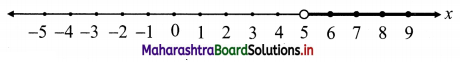(ii) x ≥ 5
Solution:
x ≥ 5
Here, x takes all real values that are greater than or equal to 5
∴ Solution set represents the unbounded (semi-left closed) interval [5, ∞)
∴ the required graph of the solution set is as follows: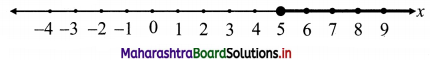(iii) x < 3
Solution:
x < 3
Here, x takes all real values that are less than 3.
∴ Solution set represents the unbounded (open) interval (-∞, 3)
∴ the required graph of the solution set is as follows: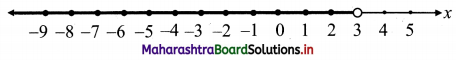(iv) x ≤ 3
Solution:
x ≤ 3
Here, x takes all real values less than and including 3
∴ Solution set represents the unbounded (semi-right closed) interval (-∞, 3]
∴ the required graph of the solution set is as follows: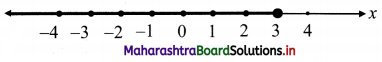(v) -4 < x < 3
Solution:
-4 < x < 3
Here, x takes all real values between -4 and 3.
∴ Solution set represents the bounded (open) interval (-4, 3)
∴ the required graph of the solution set is as follows: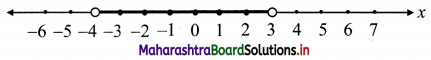(vi) -2 ≤ x < 2.5
Solution:
-2 ≤ x < 2.5
Here, x takes all values between -2 and 2.5 including -2.
∴ Solution set represents the bounded (semi-left closed) interval [-2, 2.5)
∴ the required graph of the solution set is as follows.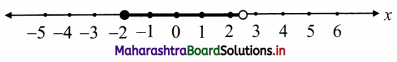(vii) -3 ≤ x ≤ 1
Solution:
-3 ≤ x ≤ 1
Here, x takes all real values between -3 and 1 including -3 and 1
∴ Solution set represents the bounded (closed) interval [-3, 1]
∴ the required graph of the solution set is as follows: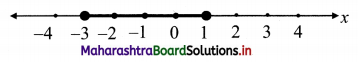(viii) |x| < 4
Solution:
|x| < 4 ⇒ -4 < x < 4
Here, x takes all real values between -4 and 4.
∴ Solution set represents bounded (open) interval (-4, 4)
∴ the required graph of the solution set is as follows: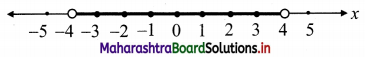(ix) |x| ≥ 3.5
Solution:
|x| ≥ 3.5 ⇒ x ≥ 3.5 or x ≤ -3.5
Here, x takes values greater than or equal to 3.5 or it takes values less than or equal to -3.5
∴ Solution set represents the unbounded (semi-left closed) interval [3.5, ∞) or the unbounded (semi-right closed) interval (-∞, -3.5]
∴ x ∈ (-∞, -3.5] ∪ [3.5, ∞)
∴ the required graph of the solution set is as follows: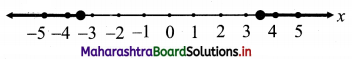Question 4.
Solve the inequations:
(i) 5x + 7 > 4 – 2x
Solution:
5x + 7 > 4 – 2x
Adding 2x on both sides, we get
7x + 7 > 4
Subtracting 7 from both sides, we get
7x > -3
Dividing by 7 on both sides, we get
∴ x > $$-\frac{3}{7}$$
i.e., x takes all real values greater than $$-\frac{3}{7}$$
∴ the solution set is ($$-\frac{3}{7}$$, ∞)

(ii) 3x + 1 ≥ 6x – 4
Solution:
3x + 1 ≥ 6x – 4
Subtracting 3x from both sides, we get
1 ≥ 3x – 4
Adding 4 on both sides, we get
5 ≥ 3x
Dividing by 3 on both sides, we get
$$\frac{5}{3}$$ ≥ x
i.e., x ≤ $$\frac{5}{3}$$
i.e., x takes all real values less than or equal to $$\frac{5}{3}$$.
∴ the solution set is (-∞, $$\frac{5}{3}$$](iii) 4 – 2x < 3(3 – x)
Solution:
4 – 2x < 3(3 – x)
∴ 4 – 2x < 9 – 3x
Adding 3x on both sides, we get
4 + x < 9
Subtracting 4 from both sides, we get
x < 5
i.e., x takes all real values less than 5
∴ the solution set is (-∞, 5)

(iv) $$\frac{3}{4}$$x – 6 ≤ x – 7
Solution:
$$\frac{3}{4}$$x – 6 ≤ x – 7
Multiplying by 4 on both sides, we get
3x – 24 ≤ 4x – 28
Subtracting 3x from both sides, we get
-24 ≤ x – 28
Adding 28 on both the sides, we get
∴ 4 ≤ x i.e., x ≥ 4
i.e., x takes all real values greater or equal to 4.
∴ the solution set is [4, ∞)

(v) -8 ≤ -(3x – 5) < 13
Solution:
-8 < -(3x – 5) < 13 Multiplying by -1 throughout (so inequality sign changes) 8 ≥ 3x – 5 > -13
i.e., -13 < 3x – 5 ≤ 8
Adding 5 on both the sides, we get
-8 < 3x ≤ 13
Dividing, by 3 on both sides, we get
∴ $$-\frac{8}{3}$$ < x ≤ $$\frac{13}{3}$$
i.e., x takes all real values between $$-\frac{8}{3}$$ and $$\frac{13}{3}$$ including $$\frac{13}{3}$$.
∴ the solution set is $$\left(-\frac{8}{3}, \frac{13}{3}\right]$$

(vi) -1 < 3 – $$\frac{x}{5}$$ ≤ 1
Solution:
-1 < 3 – $$\frac{x}{5}$$ ≤ 1
Subtracting 3 from both sides, we get
-4 < –$$\frac{x}{5}$$ < -2 Multiplying by -1 throughout (so inequality sign changes) ∴ 4 > $$\frac{x}{5}$$ > 2
i.e., 2 < $$\frac{x}{5}$$ < 4
Multiplying by 5 on both sides, we get
10 < x < 20
i.e., x takes all real values between 10 and 20.
∴ the solution set is (10, 20)

(vii) 2|4 – 5x| ≥ 9
Solution:
2|4 – 5x | ≥ 9
∴ |4 – 5x| ≥ $$\frac{9}{2}$$
∴ 4 – 5x ≥ $$\frac{9}{2}$$ or 4 – 5x ≤ –$$\frac{9}{2}$$ ……[|x| ≥ a implies x ≤ -a or x ≥ a]
Subtracting 4 from both sides, we get
-5x ≥ $$\frac{1}{2}$$ or -5x ≤ $$\frac{-17}{2}$$
Divide by -5 (so inequality sign changes)
∴ x ≤ $$-\frac{1}{10}$$ or x ≥ $$\frac{17}{10}$$
∴ x takes all real values less than or equal to $$-\frac{1}{10}$$
or it takes all real values greater or equal to $$\frac{17}{10}$$.
∴ the solution set is (-∞, $$-\frac{1}{10}$$] or [$$\frac{17}{10}$$, ∞)

(viii) |2x + 7| ≤ 25
Solution:
|2x + 7| < 25
∴ -25 ≤ 2x + 7 ≤ 25 …..[|x| ≤ a implies -a ≤ x ≤ a]
Subtracting 7 from both sides, we get
-32 ≤ 2x ≤ 18
Dividing by 2 on both sides, we get
-16 ≤ x ≤ 9
∴ x can take all real values between -16 and 9 including -16 and 9.
∴ the solution set is [-16, 9](ix) 2|x + 3| > 1
Solution:
2|x + 3| > 1
Dividing by 2 on both sides, we get
|x + 3| > $$\frac{1}{2}$$
∴ x + 3 < –$$\frac{1}{2}$$ or x + 3 > $$\frac{1}{2}$$ …..[|x| > a implies x < -a or x > a]
Subtracting 3 from both sides, we get
x < – 3 – $$\frac{1}{2}$$ or x > -3 + $$\frac{1}{2}$$
∴ x < $$\frac{-7}{2}$$ or x > $$\frac{-5}{2}$$
∴ x can take all real values less $$\frac{-7}{2}$$ or it can take values greater than $$\frac{-5}{2}$$.
∴ Solution set is (-∞, $$\frac{-7}{2}$$) ∪ ($$\frac{-7}{2}$$, ∞)

(x) $$\frac{x+5}{x-3}$$ < 0
Solution:
$$\frac{x+5}{x-3}$$ < 0
Since $$\frac{a}{b}$$ < 0, when a > 0 and b < 0 or a < 0 and b > 0
∴ either x + 5 > 0 and x – 3 < 0
or x + 5 < 0 and x – 3 > 0
Case I:
x + 5 > 0 and x – 3 < 0 ∴ x > -5 and x < 3
∴ -5 < x < 3
∴ solution set = (-5, 3)
Case II:
x + 5 < 0 and x – 3 > 0
∴ x < -5 and x > 3
which is not possible
∴ solution set = Φ
∴ solution set of the given inequation is (-5, 3)

(xi) $$\frac{x-2}{x+5}$$ > 0
Solution:
$$\frac{x-2}{x+5}$$ > 0
Since $$\frac{a}{b}$$ > 0,
when a > 0 and b > 0 or a < 0 and b < 0 b
∴ either x – 2 > 0 and x + 5 > 0
or x – 2 < 0 and x + 5 < 0 Case I: x – 2 > 0 and x + 5 > 0
∴ x > 2 and x > -5
∴ x > 2
∴ solution set = (2, ∞)
Case II:
x – 2 < 0 and x + 5 < 0
∴ x < 2 and x < -5
∴ x < -5
∴ solution set = (-∞, -5)
∴ the solution set of the given inequation is (-∞, -5) ∪ (2, ∞)

Question 5.
Rajiv obtained 70 and 75 marks in the first two unit tests. Find the minimum marks he should get in the third test to have an average of at least 60 marks.
Solution:
Let x1, x2, x3 denote the marks in 1st, 2nd and 3rd unit test respectively. Then
$$\frac{x_{1}+x_{2}+x_{3}}{3}$$ ≥ 60
∴ $$\frac{70+75+x_{3}}{3}$$ ≥ 60
∴ 145 + x3 ≥ 3(60)
Subtracting 145 from both sides, we get
x3 ≥ 180 – 145
∴ x3 ≥ 35
Rajiv must obtain a minimum of 35 marks to maintain an average of at least 60 marks.

Question 6.
To receive Grade ‘A’ in a course, one must obtain an average of 90 marks or more in five examinations (each of 100 marks). If Sunita’s marks in the first four examinations are 87, 92, 94, and 95, find the minimum marks that Sunita must obtain in the fifth examination to get a grade ‘A’ in the course.
Solution:
Let x1, x2, x3, x4, x5 denote the marks in five examinations. Then
$$\frac{x_{1}+x_{2}+x_{3}+x_{4}+x_{5}}{5}$$ ≥ 90
∴ $$\frac{87+92+94+95+x_{5}}{5}$$ ≥ 90
∴ 368 + x5 ≥ 450
Subtracting 368 from both sides, we get
∴ x5 ≥ 82
Sunita must obtain a minimum of 82 marks in the 5th examination to get a grade of A.Question 7.
Find all pairs of consecutive odd positive integers, both of which are smaller than 10 such that their sum is more than 11.
Solution:
Let two consecutive positive integers be 2n – 1, 2n + 1 where n ≥ 1 ∈ Z,
Given that 2n – 1 < 10 and 2n + 1 < 10
∴ 2n < 11 and 2n < 9
∴ 2n < 9
∴ n < $$\frac{9}{2}$$ …..(i) Also, (2n – 1) + (2n + 1) > 11
∴ 4n > 11
∴ n > $$\frac{11}{4}$$ …….(ii)
From (i) and (ii)
$$\frac{11}{4}<n<\frac{9}{2}$$ Since, n is an integer,
∴ n = 3, 4
n = 3 gives 2n – 1 = 5, 2n + 1 = 7
and n = 4 gives 2n – 1 = 7, 2n + 1 = 9
∴ The pairs of positive consecutive integers are (5, 7) and (7, 9).

Question 8.
Find all pairs of consecutive even positive integers, both of which are larger than 5 such that their sum is less than 23.
Solution:
Let 2n, 2n + 2 be two positive consecutive integers where n ≥ 1 ∈ Z.
Given that 2n > 5 and 2n + 2 > 5
∴ n > $$\frac{5}{2}$$ and 2n > 3
∴ n > $$\frac{5}{2}$$ and n > $$\frac{3}{2}$$
∴ n > $$\frac{5}{2}$$ ……(i)
Also (2n) + (2n + 2) < 23
∴ 4n + 2 < 23
∴ 4n < 21
∴ n < $$\frac{21}{4}$$ ……(ii)
From (i) and (ii)
$$\frac{5}{2}<n<\frac{21}{4}$$ and n is an integer.
∴ n = 3, 4, 5
n = 3 gives 2n = 6, 2n + 2 = 8
n = 4 gives 2n = 8, 2n + 2 = 10
n = 5 gives 2n = 10, 2n + 2 = 12
∴ The pairs of positive even consecutive integers are (6, 8) (8, 10), (10, 12)Question 9.
The longest side of a triangle is twice the shortest side and the third side is 2 cm longer than the shortest side. If the perimeter of the triangle is more than 166 cm then find the minimum integer length of the shortest side.
Solution:
Let the shortest side be x.
Then longest side length = 2x
and third side length = x + 2
Perimeter = x + 2x + x + 2 = 4x +2
Given, perimeter > 166
∴ 4x + 2 > 166
∴ 4x > 164
∴ x > 41
∴ Minimum integer length of shortest side is 42 cm.